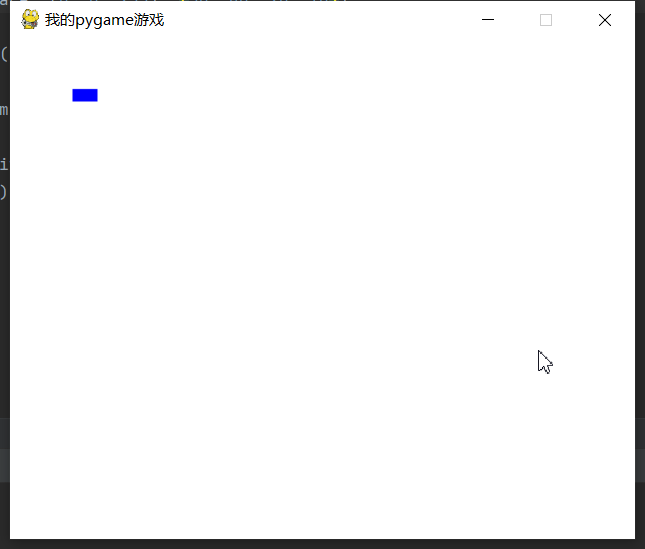# pygame基础教程

## pygame简介

pygame可以实现python游戏的一个基础包。

## pygame实现窗口

pygame.init()

surface = pygame.display.set_mode((500, 400), 0, 32)

pygame.display.set_caption(“我的pygame游戏”)

pygame.display,set_caption设置窗口的标题

``````import pygame, sys
from pygame.locals import *

pygame.init()

surface = pygame.display.set_mode((500, 400), 0, 32)
pygame.display.set_caption("我的pygame游戏")

while True:
for event in pygame.event.get():
if event.type == QUIT:
pygame.quit()
sys.exit()
``````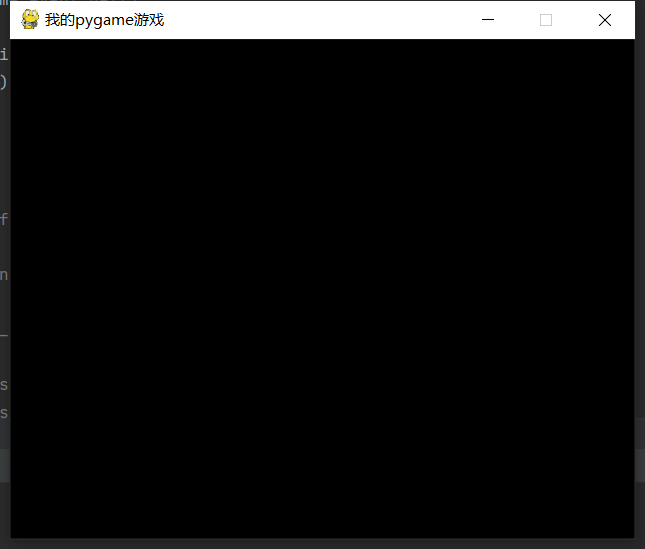## 设置屏幕背景色

``````# 设置背景颜色
surface.fill((255, 255, 255))
# 更新屏幕
pygame.display.update()
``````## 添加文字

``````# 获取字体对象
basicFont = pygame.font.SysFont(None, 40)
# surface对象
text = basicFont.render('秀儿', True, (255,255,255), (0,255,0))
# 设置文本位置
textRect = text.get_rect()

textRect.centerx = surface.get_rect().centerx
textRect.centery = surface.get_rect().centery
# 将渲染的surface对象更新到屏幕上
surface.blit(text,textRect)
``````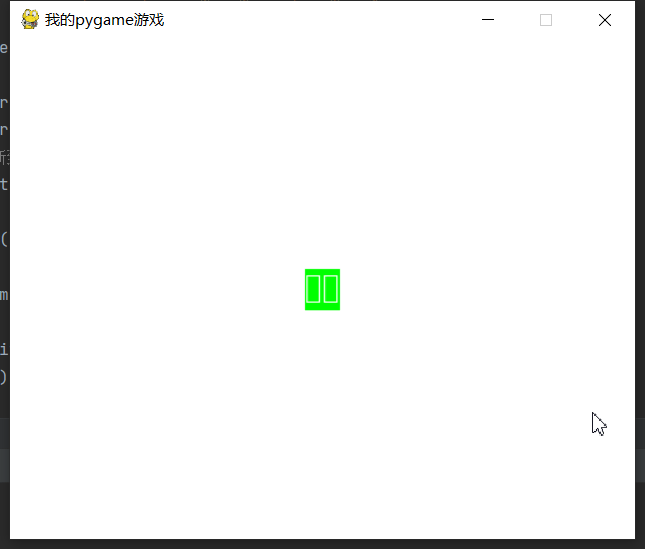``````# 获取当前系统字体
fonts = pygame.font.get_fonts()
print(fonts)
``````

``````import pygame,sys
from pygame.locals import *

pygame.init()

surface = pygame.display.set_mode((500, 400), 0, 32)
pygame.display.set_caption("我的pygame游戏")
surface.fill((255, 255, 255))

# 获取字体对象
basicFont = pygame.font.SysFont("方正粗黑宋简体", 48)
# surface对象
text = basicFont.render('秀儿', True, (255,255,255), (0,255,0))
# 设置文本位置
textRect = text.get_rect()

textRect.centerx = surface.get_rect().centerx
textRect.centery = surface.get_rect().centery
# 将渲染的surface对象更新到屏幕上
surface.blit(text,textRect)

pygame.display.update()
while True:
for event in pygame.event.get():
if event.type == QUIT:
pygame.quit()
sys.exit()
``````

## 绘制多边形

polygon 来绘制多边形，第一个参数是屏幕对象，第二个是颜色，第三个是用点串连的一个元组，最后一个点有和第一个是一致的

``````import pygame,sys
from pygame.locals import *

pygame.init()

surface = pygame.display.set_mode((500, 400), 0, 32)
pygame.display.set_caption("我的pygame游戏")
surface.fill((255, 255, 255))

pygame.draw.polygon(surface, (0, 0, 255), ((50, 40), (100, 100), (120, 80), (50, 40)))

pygame.display.update()
while True:
for event in pygame.event.get():
if event.type == QUIT:
pygame.quit()
sys.exit()
``````## 绘制直线

line方法，第一个参数是屏幕对象，之后是颜色和两个点，最后一个参数是线条宽度

``````pygame.draw.line(surface, (0, 0, 255), (50, 40), (100, 100), 10)
``````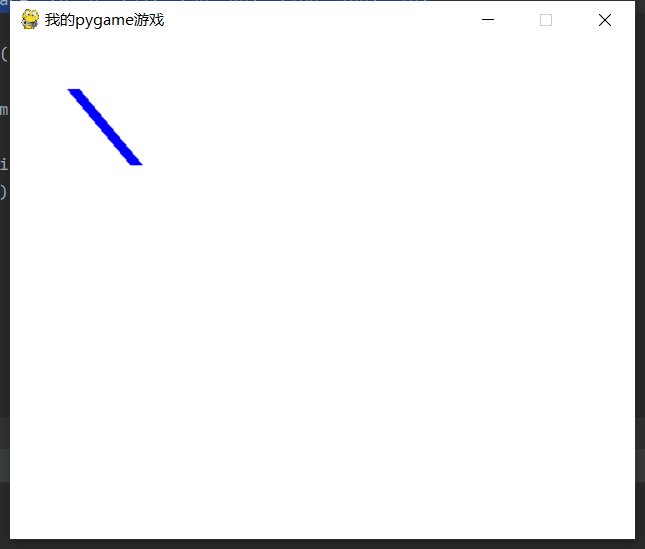## 绘制圆形

circle用来绘制圆形，第一个参数和第二个参数是屏幕对象和颜色，之后是圆心和半径，最后一个表示宽度，如果设置为0，则是一个实园。

``````pygame.draw.circle(surface, (0, 0, 255), (50, 40), 20, 10)
``````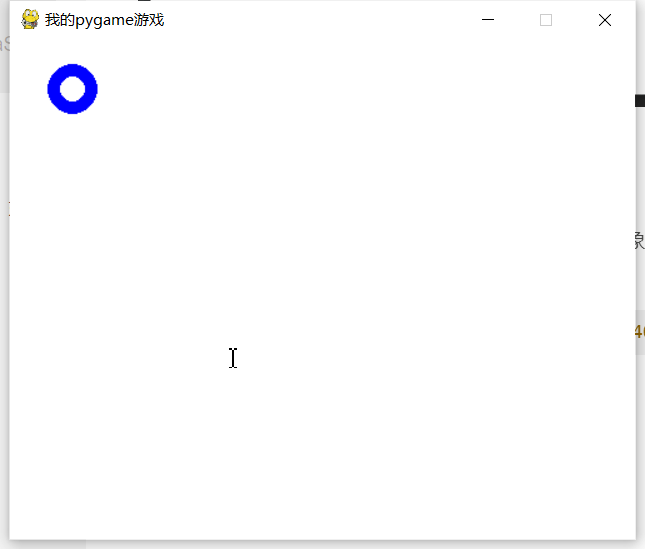## 绘制椭圆

``````pygame.draw.ellipse(surface, (0, 0, 255), (50, 40, 20, 10), 2)
``````## 绘制矩形

rect来绘制矩形，第一个和第二个参数同上，第三个参数分别制定左上角和右下角

``````pygame.draw.rect(surface, (0, 0, 255), (50, 40, 20, 10))
``````